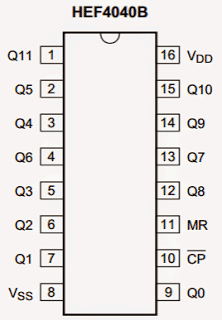Home » Electronic Components » IC 4040 Datasheet, Pinout, ApplicationIC 4040 Datasheet, Pinout, Application

The IC 4040 is technically a 12-stage binary ripple counter chip, in simple words a device which will produce a calculated delayed frequency output in response to every pulse applied at its clock input. This delay is incremented at the rate of 2^(n) where n is the pinout order in the sequence of its outputs.

Main Technical Specifications

The main features and specifications of the IC may be understood as follows:

Fully buffered 12 outputs which divide the input clocks at the rate 2^(n) where n = the pinout order starting from Q0 until Q11.

The above sequencing of the outputs happens in response to every falling edge of the clock applied at its clock input CP pinout. The IC will respond even to a relatively slow falling clock pulse as effectively.

A single asynchronous master reset (MR) input which resets all outputs to zero when a high logic is applied, whereas a constant low logic enables the IC to stay active.

The IC becomes fully operational with Vdd as low as of 3V and sustains a constant operational characteristic even at voltages around 15V.

Let's examine the parameters which shouldn't be exceeded for the IC 4040

• Supply Voltage (Vdd) = Typically between 3V and 15V, 18V being the maximum limit.
• Input Voltage (Vi) = The voltage that may be applied at the inputs such as CP, MR etc should be typically below Vdd or at the most = Vdd + 0.5V
• Optimal Operating Current Requirement = 50mA since so many outputs are involved and each output

Pinout DetailsThe diagram above depicts the pinout configuration of the IC 4040, they may be evaluated as given under:

Pinouts Q0 to Q11 are the outputs of the IC.

1. Vss is the ground pin.
2. Vdd is the positive pin.
3. MR is the reset pinout
4. CP is the clock input.

Timing Sequence

Now let's analyze the output timing sequence of the IC 4040. As shown in the following diagram, we are able to see and understand the following details:

As long as the MR input is high, the IC outputs produce no response. As soon as it goes low, the IC starts responding and counting the input clock at the CP input.

The first output pin Q0 goes high after 2^(n) clock at CP, that's = 2^(0) = 1, meaning Q0 becomes high at the falling edge of the first pulse and goes low in response to the falling edge of the subsequent clock and so on.
Similarly Q1 goes high after 2^(1) = 2, meaning it goes high as soon as a falling edge of the second clock is detected and goes low at the falling edge of the 4th subsequent clock and so on.

Identically Q2 goes high and low after 2^(2) = 4th clock's falling edges, and so on.

The above sequence is continued until Q11, in response to the sustained clock inputs at CP .

It means if suppose the CP is clocked with a 1Hz pulse, Q11 would go high after 2^11 seconds or after 2048 seconds that's equal to  34 minutes approximately, just imagine the range of delay that you can achieve by simply increasing the clock input by seconds or perhaps by minutes.Application Hints

From the above detailed analysis of the IC 4040 datasheet we can conclude that the IC is typically suited for all application which involve frequency division requirements or delayed time period generation requirements.

Therefore it could become specifically suited for frequency divider circuit applications, long duration timers, flashers and other such similar applications.

SHARING IS CARING!